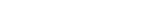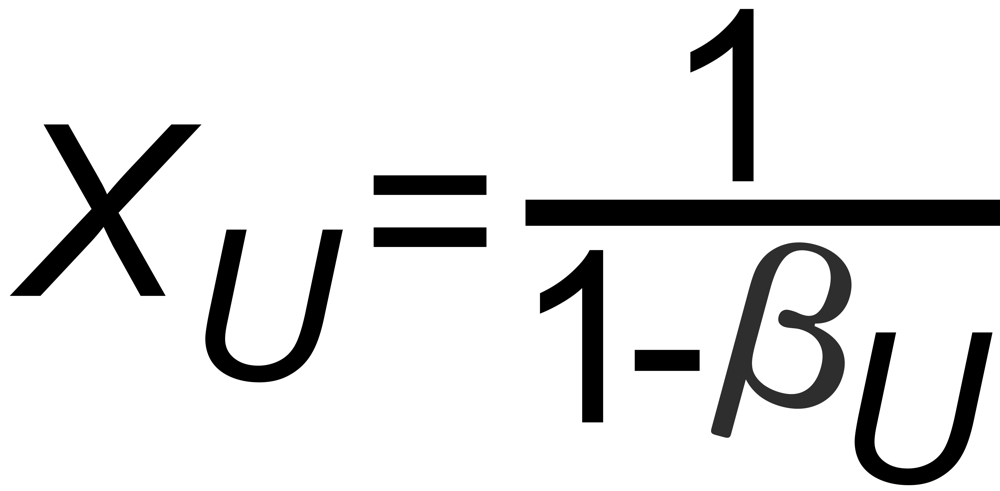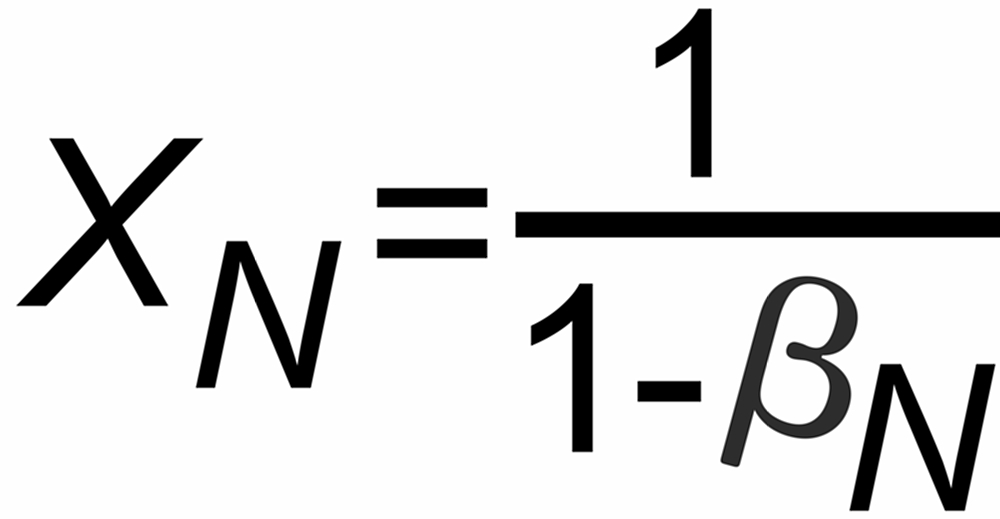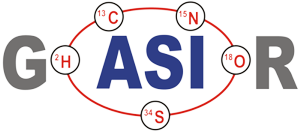,For details of the derivation of this (and all following) equation(s), see K. Habfast, Int. J. Mass Spectr. 176 (1998) 133
The root (RU) of the following equation must be iteratively calculated:
The q coefficients of the linear and power law are not only time, but also mass dependant. Theoretically , we hence must obey similar constraints as with the non iterative Rayleigh law.
1
5
6
Footnote
Description(
)Dr. Karleugen Habfast: Theory of Fractionation Correction - Footnotes in use / Description